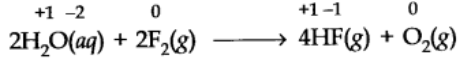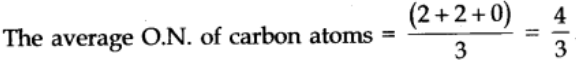• It is an imaginary or apparent charge developed over atom of an element when it goes from its elemental free state to combined state in molecules.
• It is calculated on basis of an arbitrary set of rules.
• It is a relative charge in a particular bonded state.
• In order to keep track of electron-shifts in chemical reactions involving formation of compounds, a more practical method of using oxidation number has been developed.
• In this method, it is always assumed that there is a complete transfer of electron from a less electronegative atom to a more electronegative atom.

# Rules for Assigning Oxidation Numbers

• The oxidation number of an element in its elementary form is zero.
e.g., H2, 02, Netc. have oxidation number equal to zero.
• In a single monoatomic ion, the oxidation number is equal to the charge on the ion.

e.g., Na+ ion has oxidation number of +1 and Mg2+ ion has +2.

• Oxygen has oxidation number -2 in its compounds. However, there are some exceptions.

Compounds such as peroxides. Na202, H202
oxidation number of Oxygen = – 1 In OF2
O.N. of oxygen = +2 02F2
O.N. of oxygen = +1

• In non-metallic compounds of hydrogen like HCl, H2S, H2O
• oxidation number of Hydrogen = + 1 but
• in metal hydrides oxidation number of Hydrogen = -1

[LiH, NaH, CaH2 etc.]

• In compounds of metals and non-metals,
• metals have positive oxidation number
• non-metals have negative oxidation number.

e.g., In NaCl. Na has +1 oxidation number while chlorine has -1.

• If in a compound there are two non-metallic atoms the atoms with high electronegativity is assigned negative oxidation number while other atoms have positive oxidation number.
• The algebraic sum of the oxidation number of all atoms in a compound is equal to zero.
• In poly-atomic ion the sum of the oxidation no. of all the atoms in the ion is equal to the net charge on the ion.

e.g., In (C03)2—Sum of carbon atoms and three oxygen atoms is equal to -2.
Fluorine (F2) is so highly reactive non-metal that it displaces oxygen from water. e.g.,# Fractional Oxidation Numbers

Elements as such do not have any fractional oxidation numbers. When the same element are involved in different bonding in a species, their actual oxidation states are whole numbers but an average of these is fractional.
For example, In C302Fractional O.N. of a particular element can be calculated only if we know about the structure of the compound or in which it is present.